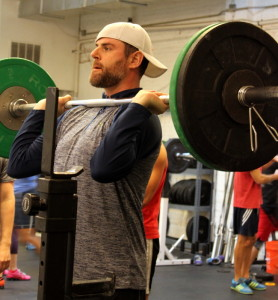## Friday, January 9, 2015

### Friday, January 9, 2015Complete 10 minutes, every minute, on-the-minute of 1x high hang snatch + 1x snatch balance.

then, with a continuously running clock complete one ring pushup the first minute, two ring pushups the second minute, three ring pushups the third minute, etc.—continuing as long as you are able.

then, with a continuously running clock complete one strict chin-up the first minute, two strict chin-ups the second minute, three strict chin-ups the third minute, etc.—continuing as long as you are able.

### 1 Comment

1.–Scores–
Brian G.: 85-lbs., 9 rounds + 1, 7 rounds + 4
Kate H.: 55-lbs., 8 rounds + 5, 7 rounds + 7 (sub: knee pushup, strict bodyrow)
Dave R.: 155-lbs., 12 rounds + 11, 9 rounds + 8
Matthew W.: 115-lbs., 13 rounds + 10, 10 rounds + 6 (started at 5)
John L.: 85-lbs., 11 rounds + 10, 4 rounds + 3 (sub: 2x high hang snatch, pushup, jum-stretch strict pull-up, blue)
Blaine P.: 115-lbs., 14 rounds + 4, 10 rounds + 4 (sub: 2x high hang snatch, started at 5)
Kevin M.: 45-lbs., 9 rounds + 9, 5 rounds + 5
Joe T.: 45-lbs., 15 rounds + 14, 10 rounds + 6 (started at 5)
Judy G.: 65-lbs., 11 rounds + 8, 4 rounds + 4 (sub: pushup)
Diana D.: 35-lbs., 10 rounds + 5, 5 rounds + 3 (sub: pushup, jump-stretch strict pull-up, red)
Amy S.: 85-lbs., 11 rounds + 9, 8 rounds + 4
Zaid A.: 45-lbs., 6 rounds + 6, 7 rounds + 2 (sub: pushup, jump-stretch strict pull-up, green)
James W.: 65-lbs., 7 rounds + 1, 9 rounds + 5 (sub: pushup, jump-stretch strict pull-up, green)
Kyle S.: 65-lbs., 12 rounds, 10 rounds + 1 (started at 3)
Francisco L.: 65-lbs., 9 rounds + 6, 5 rounds + 3
Bill M.: 25-lbs., 10 rounds + 6, 8 rounds + 4
Ken F.: 25-lbs., 5 rounds + 5, 6 rounds + 2
James H.: 105-lbs., 15 rounds + 11, 10 rounds + 5 (sub: 2x snatch balance)
Abby K.: 45-lbs., 10 rounds, 9 rounds + 6 (sub: incline pushup, strict bodyrow)
Buffy M.: 45-lbs., 11 rounds + 8, 6 rounds (sub: pushup, jump-stretch strict pull-up, red/blue)
Tracey S.: 45-lbs., 6 rounds + 2, 9 rounds + 9 (sub: incline pushup, strict bodyrow)
Alex M.: 105-lbs., 8 rounds + 4, 5 rounds + 4
Mayra C.: 55-lbs., 10 rounds, 8 rounds + 4 (sub: pushup, jump-stretch strict pull-up, blue)
Anna G.: 35-lbs., 6 rounds + 5, 8 rounds + 5 (sub: power snatch + ohs, incline pushup, strict bodyrow)
Ryan L.: 115-lbs., 7 rounds + 5, 6 rounds + 4
Rob C.: 65-lbs., 13 rounds + 10, 7 rounds + 7 (started at 3 for pull-ups)
Rebekka E.: 55-lbs., 7 rounds + 2, 3 rounds + 3
Melody F.: 95-lbs., 11 rounds + 11, 9 rounds + 6 (started at 3)
Ralph B.: 80-lbs., 12 rounds + 12, 9 rounds + 4 (stated at 3)
Adrian P.: 80-lbs., 12 rounds + 9, 8 rounds + 5
Dave B.: 50-lbs., 12 rounds + 11, 7 rounds + 4
Teal B.: 15-lbs., 9 rounds + 7, 10 rounds (sub: 30″ v-hold, pushup)
Lisa C.: 35-lbs., 9 rounds + 6, 6 rounds + 3 (sub: power snatch + ohs, pushup, jump-stretch strict pull-up, red)
Kate D.: 125-lbs., 12 rounds + 12, 8 rounds + 5 (started at 3)
Drew P.: 135-lbs., 14 rounds + 5, 9 rounds + 9
Alissa G.: 85-lbs., 11 rounds + 7, 8 rounds + 6 (sub: front squat, 3RM, started at 3 for pull-ups)
David G.: 55-lbs., 11 rounds + 10, 5 rounds + 4 (sub: pushup, jump-stretch strict pull-up, blue)
Shawn J.: 85-lbs., 7 rounds + 17, 6 rounds + 4
Hari P.: 50-lbs., 10 rounds + 8, 7 rounds + 3
Dat D.: 95-lbs., 19 rounds + 11, 9 rounds + 3 (started at 3)
Michael F.: (sub: strict pull-up practice)